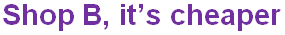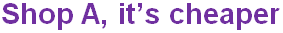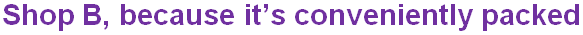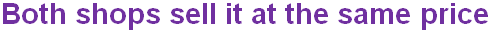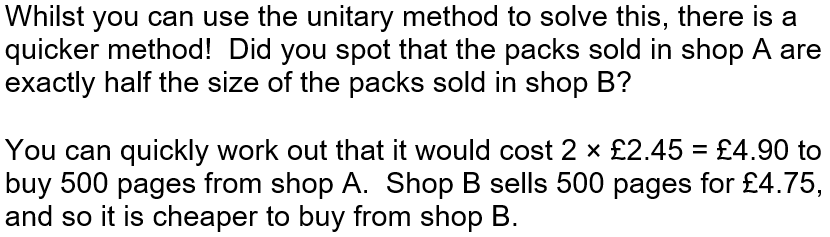In question 3, Sally is in Greece and needs your help with exchanging her currency.

# Unitary Method of Solution (F)

The Unitary Method sounds like it might be complicated but it’s not! It’s a very useful way to solve problems involving ratio and proportion, so once you’ve mastered the technique in this GCSE Maths quiz have a go at the Ratio and Proportion quiz too!

The method involves scaling down one of the variables to a single unit, i.e. 1. Once we know the value of 1 unit, the value of multiple units can be found by multiplying. This will make more sense when it is explained using an example. If 12 tins of paint weigh 30kg, how much will 5 tins weigh? The first step in solving this is to find what ONE tin weighs. This will be 30/12 so 2.5kg. Scaling this back up for 5 tins gives 5 * 2.5 = 12.5kg.

The unitary method is also useful for working out which deal will give better value for money.

A 300ml can of juice costs 85p, while a 500ml bottle of the same juice costs £1.20. Which is better value?

As you are going to be in a shop when faced with this problem, you probably won’t have your calculator on you to divide by 300 and 500! Rather than working out what 1ml will cost, it is just as valid to work out the cost of 100ml for each option. For the can, 100ml will cost (85/3) 28.33 pence, whilst for the bottle 100ml will cost (120/5) 24 pence. You should be able to perform these calculations in your head: with practise, you will improve! We can see in this comparison that the bottle is better value.

Question 1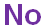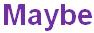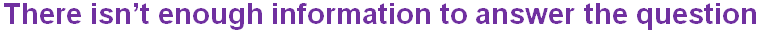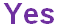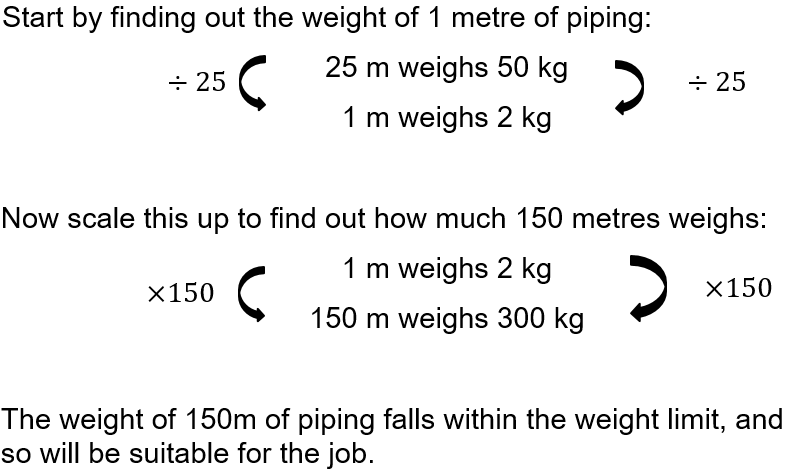Question 2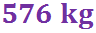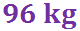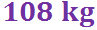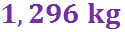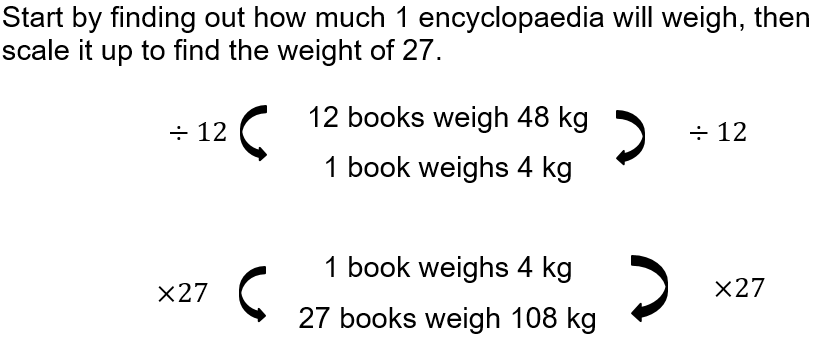Question 3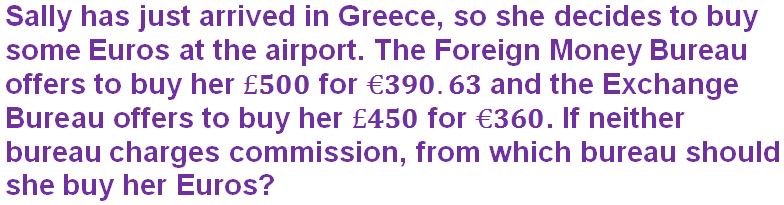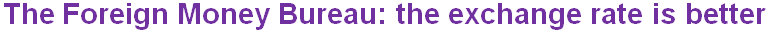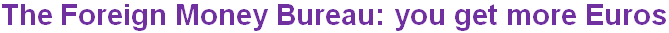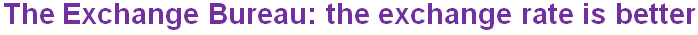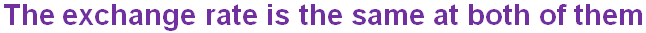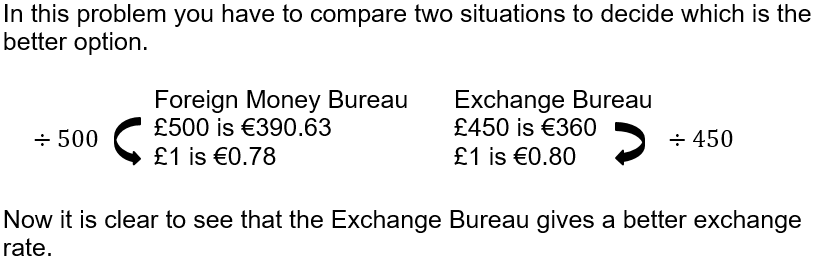Question 4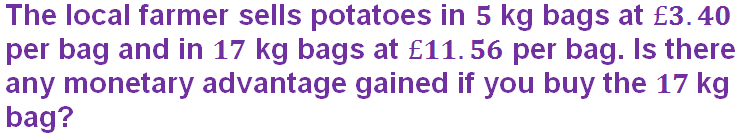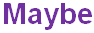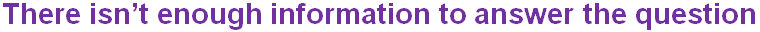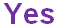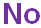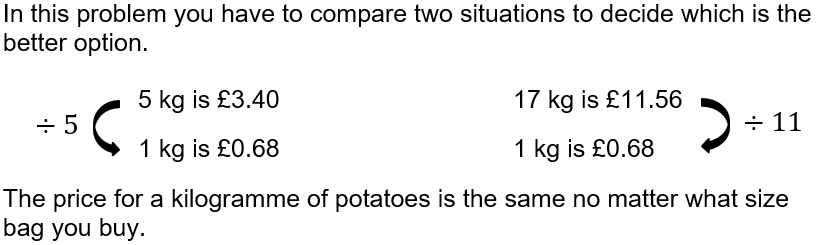Question 5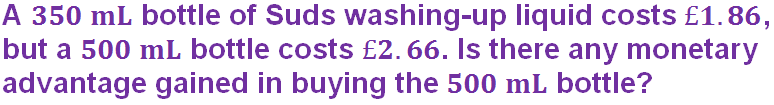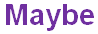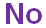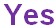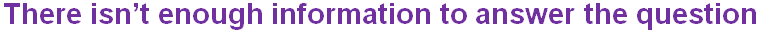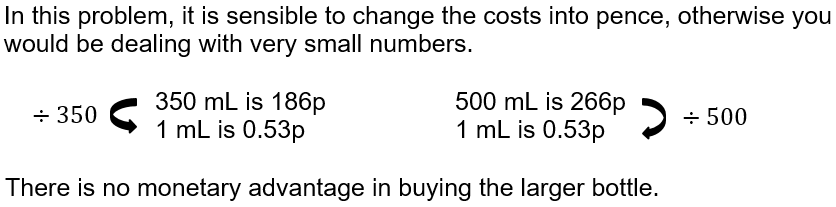Question 6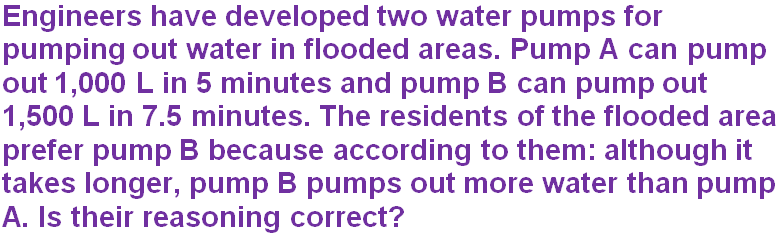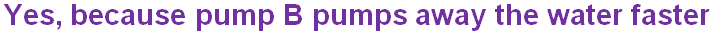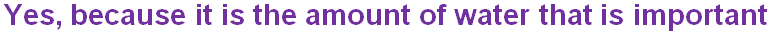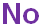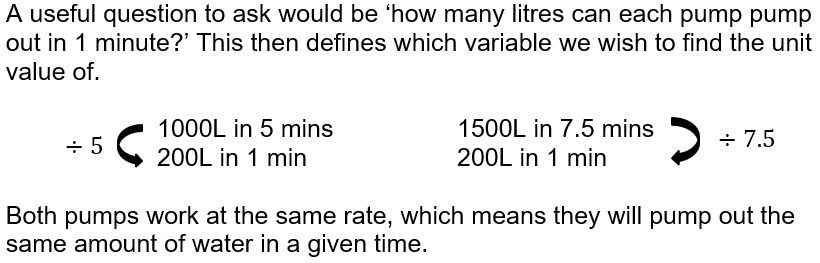Question 7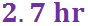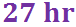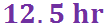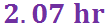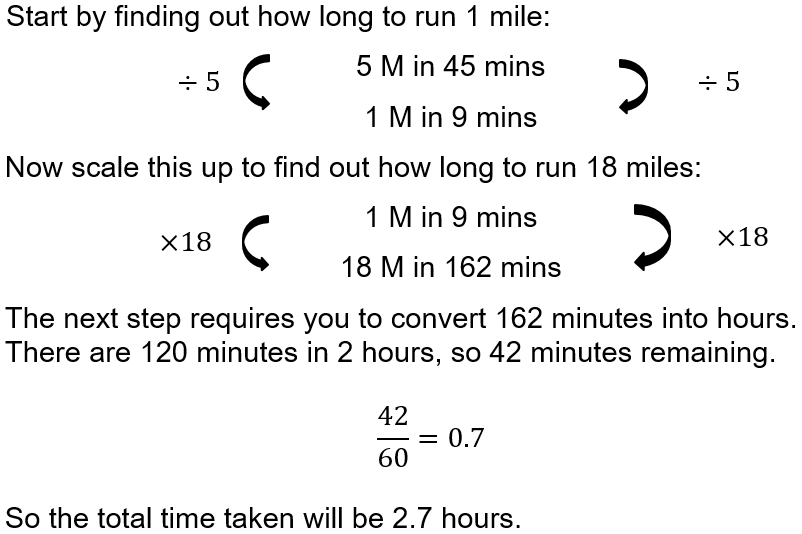Question 8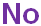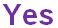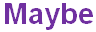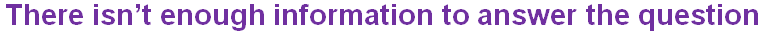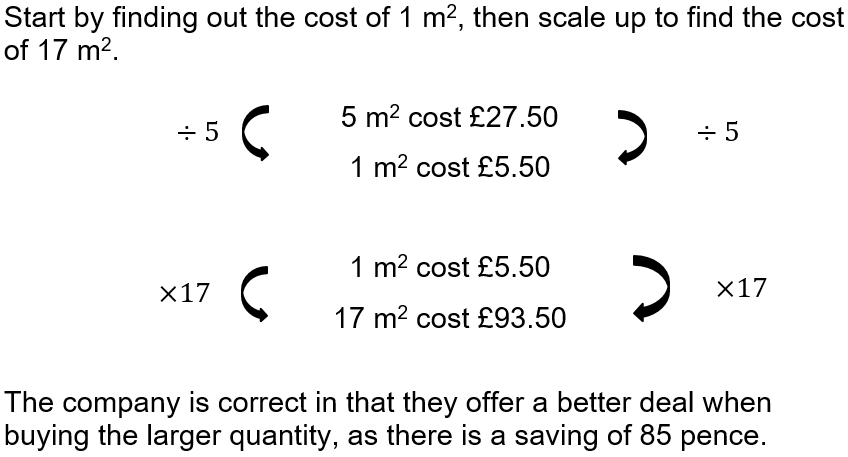Question 9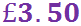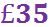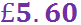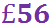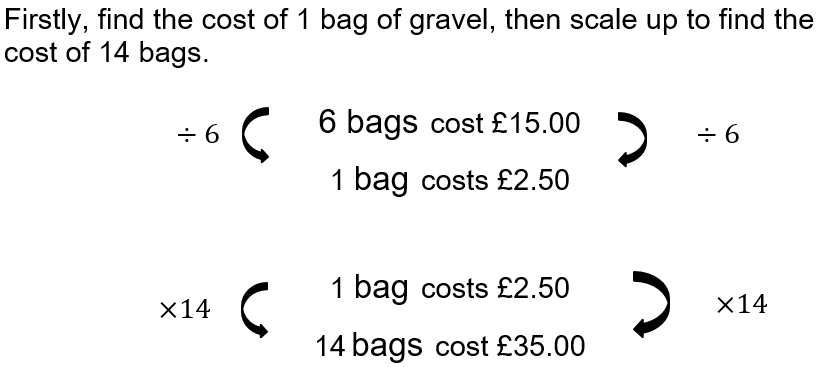Question 10